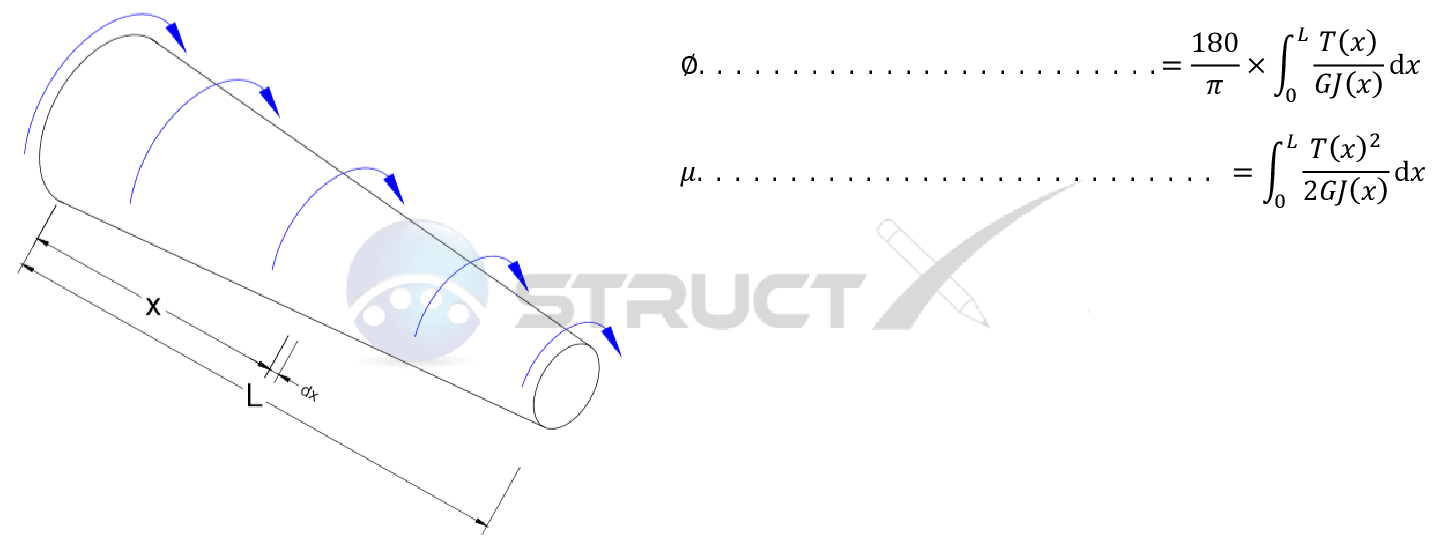# Notation and Units

## Metric and Imperial Units

The above formulas may be used with both imperial and metric units. As with all calculations care must be taken to keep consistent units throughout with examples of units which should be adopted listed below:

# Notation

• L = length under consideration, in or mm
• G = shear modulus or modulus of rigidity, psi or MPa
• J = polar moment of inertia, in4 or mm4
• r = radial distance from centre to point of interest, in or mm
• T = applied or resulting torsion, lb.in or Nmm
• x = distance to point of interest, in or mm
• θ = angle of twist, degrees
• µ = strain energy, ib.in or Nmm